NBody Simulation

## Introduction

The goal of this project is to give you a crash course in Java. Since CS61B is not intended to be a course about Java, we will be moving quickly, learning fundamental aspects of the Java language in just four weeks.

Before starting this project, you should have:

You do not need to fully understand the contents of lecture 2 to begin this assignment. Indeed, the main purpose of this project is to help you build some comfort with the material in that lecture.

Unlike later projects, this assignment has a great deal of scaffolding. Future assignments will require significantly more independence. This project should be entirely your own work, i.e. you may not work closely with a partner or a group.

However, you’re welcome to discuss the project with your classmates as described in the rules described in the Course Info page.

Your goal for this project is to write a program simulating the motion of N objects in a plane, accounting for the gravitational forces mutually affecting each object as demonstrated by Sir Isaac Newton’s Law of Universal Gravitation.

Ultimately, you will be creating a program NBody.java that draws an animation of bodies floating around in space tugging on each other with the power of gravity.

If you run into problems, be sure to check out the FAQ section before posting to Ed Discussions. We’ll keep this section updated as questions arise during the assignment. Always try googling before asking questions on Ed. Knowing how to find what you want on Google is a valuable skill. However, know when to yield! If you start getting frustrated with your search attempts, then turn to Ed. Be sure to check out our Ed Guide and Ed Policies.

## Getting the Skeleton Files

Before proceeding, make sure you have completed lab1, and if you are working on your own computer, that you have completed lab1setup to set up your computer.

Now we’ll make sure you have the latest copy of the skeleton files with git pull skeleton master. Note: This command may result in a “merge”. You’ll know a merge has occurred if a text editor automatically opens. If this happens, see the Skeleton Merge Guide.

## The Body Class and Its Constructor

You’ll start by creating a Body class. In your favorite text editor, create a new file called Body.java. If you haven’t picked a text editor, we recommend Sublime Text. Remember that your .java files should have the same name as the class it contains.

Note: You should not use IntelliJ for this project! If you don’t know what IntelliJ is, good, that’s what we’ll be talking about in lab 2 (next week).

Begin by creating a basic version of the Body class with the following 6 instance variables:

• double xxPos: Its current x position
• double yyPos: Its current y position
• double xxVel: Its current velocity in the x direction
• double yyVel: Its current velocity in the y direction
• double mass: Its mass
• String imgFileName: The name of the file that corresponds to the image that depicts the body (for example, jupiter.gif)

Your instance variables must be named exactly as above, and they must be explicitly set to public via the public keyword. The reason we call them by double letters, e.g. xxPos rather than xPos is to reduce the chance of typos. In past semesters, students have accidentally pressed x when they meant y, and this has caused significant debugging hassle. After adding the 6 instance variables above, add in two Body constructors that can initialize an instance of the Body class. Later on, an instance of the Body class can represent a planet, star, or various objects in this universe. The signature of the first constructor should be:

public Body(double xP, double yP, double xV,
double yV, double m, String img)


Note: We have given parameter names that are different than the corresponding instance variable name. If you insist on making the parameter names the same as the instance variable names for aesthetic reasons, make sure to use the "this" keyword appropriately (mentioned only briefly in lecture).

The second constructor should take in a Body object and initialize an identical Body object (i.e. a copy). The signature of the second constructor should be:

public Body(Body b)


Your Body class should NOT have a main method, because we’ll never run the Body class directly (i.e. we will never run java Body). Also, all methods should be non-static.

All of the numbers for this project will be doubles. We’ll talk more aobut what a double is later in the course, but for now, think of it is a real number, e.g. double x = 3.5. In addition, all instance variables and methods will be declared using the public keyword.

Once you have filled in the constructors, you can test it out by compiling your Body.java file and the TestBodyConstructor.java file we have provided.

You can compile with the command:

javac Body.java TestBodyConstructor.java


Don’t worry if you get warnings about deprecation when compiling for this project.

You can run our provided test with the command

java TestBodyConstructor


If you pass this test, you’re ready to move on to the next step. Do not proceed until you have passed this test.

## Understanding the Physics

In this section, we’re not going to do any programming. Instead, we’re going to review some basic physics that we’ll need to understand in order to continue with the project. At the end of this section you should complete the exercise labeled “Samh, Aegir, and, Rocinante”. There is no way to submit this work, but make sure you can understand it before you proceed to the following section.

Our Body objects will obey the laws of Newtonian physics.

### Pairwise Force

Newton’s law of universal gravitation asserts that the strength of the gravitational force between two particles is given by the product of their masses divided by the square of the distance between them, scaled by the gravitational constant $G = 6.67 \cdot 10^{-11} \dfrac{\text{Nm}^2}{\text{kg}^2}$. The gravitational force exerted on a particle is along the straight line between them (we are ignoring here strange effects like the curvature of space). Since we are using Cartesian coordinates to represent the position of a particle, it is convenient to break up the force into its $x$- and $y$-components ($F_x$, $F_y$). The relevant equations are shown below. We have not derived these equations, and you should just trust us.

• $F = \dfrac{G \cdot m_1 \cdot m_2}{r^2}$
• $r^2 = dx^2 + dy^2$
• $F_x = \dfrac{F \cdot dx}{r}$
• $F_y = \dfrac{F \cdot dy}{r}$

Note that force is a vector (i.e., it has direction). In particular, be aware that dx and dy are signed (positive or negative).

Consider a small example consisting of two celestial objects: Saturn and the Sun. Suppose the Sun is at coordinates $(1.0 \cdot 10^{12}, 2.0 \cdot 10^{11})$ and Saturn is at coordinates $(2.3 \cdot 10^{12}, 9.5 \cdot 10^{11})$. Assume that the Sun’s mass is $2.0 \cdot 10^{30} \text{ kg}$ and Saturn’s mass is $6.0 \cdot 10^{26} \text{ kg}$. Here’s a diagram of this simple solar system:Let’s run through some sample calculations. First let’s compute $F_1$, the force that Saturn exerts on the Sun. We’ll begin by calculating $r$, which we’ve already expressed above in terms of $dx$ and $dy$. Since we’re calculating the force exerted by Saturn, $dx$ is Saturn’s $x$-position minus Sun’s $x$-position, which is $1.3 \times 10^{12} \text{ m}$. Similarly, $dy$ is $7.5 \cdot 10^{11} \text{ m}$.

So, $r^2 = dx^2 + dy^2 = (1.3 \cdot 10^{12})^2 + (7.5 \cdot 10^{11})^2$. Solving for $r$ gives us $1.5 \cdot 10^{12} \text{ m}$. Now that we have $r$, computation of $F$ is straightforward:

$F = \dfrac{G \cdot (2.0 \cdot 10^{30} \text{ kg}) \cdot (6.0 \cdot 10^{26} \text{kg})}{(1.5 \cdot 10^{12} \text{ m})^2} = 3.6 \cdot 10^{22} \text{ N}$

Note that the magnitudes of the forces that Saturn and the Sun exert on one another are equal; that is, $|F| = |F_1| = |F_2|$. Now that we’ve computed the pairwise force on the Sun, let’s compute the $x$- and $y$-components of this force, denoted with $F_{1,x}$ and $F_{1, y}$, respectively. Recall that $dx$ is $1.3 \cdot 10^{12}$ meters and $dy$ is $7.5 \cdot 10^{11}$ meters. So,

• $F_{1,x} = \dfrac{F_1 \cdot (1.3 \cdot 10^{12} \text{ m})}{1.5 \cdot 10^{12} \text{ m}} = 3.1 \cdot 10^{22} \text{ N}$
• $F_{1, y} = \dfrac{F_1 \cdot (7.5 \cdot 10^{11} \text{ m})}{1.5 \cdot 10^{12} \text{ m}} = 1.8 \cdot 10^{22} \text{ N}$

Note that the sign of $dx$ and $dy$ is important! Here, $dx$ and $dy$ were both positive, resulting in positive values for $F_{1, x}$ and $F_{1, y}$. This makes sense if you look at the diagram: Saturn will exert a force that pulls the Sun to the right (positive $F_{1, x}$ ) and up (positive $F_{1, y}$).

Next, let’s compute the x and y-components of the force that the Sun exerts on Saturn. The values of $dx$ and $dy$ are negated here, because we’re now measuring the displacement of the Sun relative to Saturn. Again, you can verify that the signs should be negative by looking at the diagram: the Sun will pull Saturn to the left (negative $dx$) and down (negative $dy$).

• $F_{2, x} = \dfrac{F_2 \cdot (-1.3 \cdot 10^{12} \text{ m})}{1.5 \cdot 10^{12} \text{ m}} = -3.1 \cdot 10^{22} \text{ N}$
• $F_{2, y} = \dfrac{F_2 \cdot(-7.5 \cdot 10^{11} \text{ m})}{1.5 \cdot 10^{12} \text{ m}} = -1.8 \cdot 10^{22} \text{ N}$

### Net Forces

The principle of superposition says that the net force acting on a particle in the x- or y-direction is the sum of the pairwise forces acting on the particle in that direction.

To illustrate this idea, let’s add Neptune to the mix and calculate the net force on Saturn. Here’s a diagram illustrating the forces being exerted on Saturn in this new system: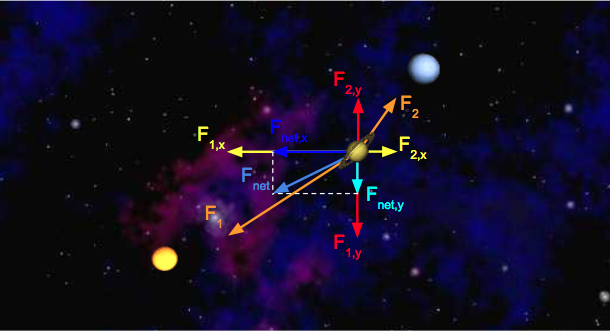We can calculate the $x$-component of the net force on Saturn by summing the $x$-components of all pairwise forces. Likewise, $F_{\text{net}, y}$ can be calculated by summing the $y$-components of all pairwise forces. Assume the forces exerted on Saturn by the Sun are the same as above, and that $F_{2,x} = 1.1 \cdot 10^{22} \text{ N}$ and $F_{2,y} = 9.0 \cdot 10^{21} \text{N}$.

• $F_{\text{net}, x} = F_{1, x} + F_{2, x} = -3.1 \cdot 10^{22} \text{ N} + 1.1 \cdot 10^{22} \text{ N} = -2.0 \cdot 10^{22} \text{ N}$
• $F_{\text{net}, y} = F_{1, y} + F_{2, y} = -1.8 \cdot 10^{22} \text{ N} + 9.0 \cdot 10^{21} \text{ N} = -9.0 \cdot 10^{21} \text {N}$

### Force and Acceleration

Newton’s second law of motion says that the accelerations in the x- and y-directions are given by:

• $a_x = \dfrac{F_x}{m}$
• $a_y = \dfrac{F_y}{m}$

For example, Saturn will experience an acceleration in the x direction of $\dfrac{F_{\text{net}, x}}{m} = \dfrac{-2.0 \cdot 10^{22} \text{ N}}{6.0 \cdot 10^{26} kg} = -2.0 \cdot 10^{-4} m/s^2$.

### Samh, Aegir, and, Rocinante

Suppose there are three bodies in space as follows:

• Samh: $x = 1, y = 0, \text{mass} = 7 \cdot 10^5$
• Aegir: $x = 3, y = 3, \text{mass} = 8 \cdot 10^5$
• Rocinante: $x = 5, y = -3, \text{mass} = 9 \cdot 10^6$

Show that $F_{\text{net}, x}$ and $F_{\text{net}, y}$ exerted on Samh are around 15.04 N and -7.69 N, respectively. If you’re stuck and can’t figure these out, see: here for $F_{\text{net}, x}$ and here for $F_{\text{net}, y}$.

## Writing the Body Class

In our program, we’ll have instances of the Body class do the job of calculating all the numbers we learned about in the previous example. We’ll write helper methods, one by one, until our Body class is complete.

#### calcDistance

Start by adding a method called calcDistance that calculates the distance between two Bodys. This method will take in a single Body and should return a double equal to the distance between the supplied body and the body that is doing the calculation, e.g.

    samh.calcDistance(rocinante);


It is up to you this time to figure out the signature of the method. Once you have completed this method, go ahead and recompile and run the next unit test to see if your code is correct.

Compile and run with:

javac Body.java TestCalcDistance.java
java TestCalcDistance


Note that in Java, there is no built-in operator that does squaring or exponentiation.

#### calcForceExertedBy

The next method that you will implement is calcForceExertedBy. The calcForceExertedBy method takes in a Body, and returns a double describing the force exerted on this body by the given body. You should be calling the calcDistance method inside this method. As an example, samh.calcForceExertedBy(rocinante) for the numbers in “Double Check Your Understanding” return $16.8084$. The force exerted by a body on itself should be 0. To compare two bodies, use the .equals method instead of ==: samh.equals(samh) (which would return true). In week 2, we will explain the difference.

Once you’ve finished calcForceExertedBy, re-compile and run the next unit test.

javac Body.java TestCalcForceExertedBy.java
java TestCalcForceExertedBy


Hint 1: Java supports scientific notation. For example, I can write double someNumber = 1.03e-7;.

#### calcForceExertedByX and calcForceExertedByY

The next two methods that you should write are calcForceExertedByX and calcForceExertedByY. Unlike the calcForceExertedBy method, which returns the total force, these two methods describe the force exerted in the X and Y directions, respectively. Remember to check your signs! Once you’ve finished, you can recompile and run the next unit test. As an example, samh.calcForceExertedByX(rocinante) in “Double Check Your Understanding” should return $13.44672$.

NOTE: Do not use Math.abs to fix sign issues with these methods. This will cause issues later when drawing planets.

javac Body.java TestCalcForceExertedByXY.java
java TestCalcForceExertedByXY


#### calcNetForceExertedByX and calcNetForceExertedByY

Write methods calcNetForceExertedByX and calcNetForceExertedByY that each take in an array of Bodys and calculates the net X and net Y force exerted by all bodies in that array upon the current Body. For example, consider the code snippet below:

Body[] allBodys = {samh, rocinante, aegir};
samh.calcNetForceExertedByX(allBodys);
samh.calcNetForceExertedByY(allBodys);


The two calls here would return the values given in “Double Check Your Understanding.”

As you implement these methods, remember that Bodys cannot exert gravitational forces on themselves! Can you think of why that is the case (hint: the universe will possibly collapse in on itself, destroying everything including you)? To avoid this problem, ignore any body in the array that is equal to the current body.

When you are done go ahead and run:

javac Body.java TestCalcNetForceExertedByXY.java
java TestCalcNetForceExertedByXY


We recommend using the “enhanced for loop” for this part of the assignment. In case you missed it in HW0, you can read more about them at this link. HW0. This is not necessary to complete the project, but it’s a nice piece of syntax with which to be familiar.

#### update

Next, you’ll add a method that determines how much the forces exerted on the body will cause that body to accelerate, and the resulting change in the body’s velocity and position in a small period of time $dt$. For example, samh.update(0.005, 10, 3) would adjust the velocity and position if an $x$-force of $10 \text{ Newtons}$ and a $y$-force of $3 \text{ Newtons}$ were applied for $0.005 \text{ seconds}$.

You must compute the movement of the Body using the following steps:

1. Calculate the acceleration using the provided $x$- and $y$-forces.
2. Calculate the new velocity by using the acceleration and current velocity. Recall that acceleration describes the change in velocity per unit time, so the new velocity is $(v_x + dt \cdot a_x, v_y + dt \cdot a_y)$.
3. Calculate the new position by using the velocity computed in step 2 and the current position. The new position is $(p_x + dt \cdot v_x, p_y + dt \cdot v_y)$.

Let’s try an example! Consider a squirrel initially at position $(0, 0)$ with a $v_x$ of $3 \dfrac{\text{m}}{\text{s}}$ and a $v_y$ of $5 \dfrac{\text{m}}{\text{s}}$. $F_{\text{net}, x}$ is $-5 \text{ N}$ and $F_{\text{net}, y}$ is $-2 \text{ N}$. Here’s a diagram of this system: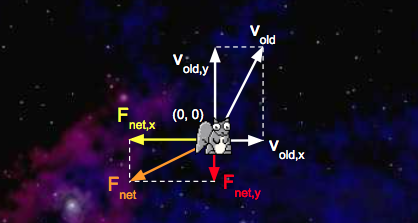We’d like to update with a time step of $1 \text{ second}$. First, we’ll calculate the squirrel’s net acceleration:

• $a_{\text{net}, x} = \dfrac{F_{\text{net}, x}}{m} = \dfrac{-5 \text{ N}}{1 \text{ kg}} = -5 \dfrac{\text{m}}{\text{s}^2}$
• $a_{\text{net}, y} = \dfrac{F_{\text{net}, y}}{m} = \dfrac{-2 \text{ N}}{1 \text{ kg}} = -2 \dfrac{\text{m}}{\text{s}^2}$

With the addition of the acceleration vectors we just calculated, our system now looks like this:Second, we’ll calculate the squirrel’s new velocity:

• $v_{\text{new}, x} = v_{\text{old}, x} + dt \cdot a_{\text{net}, x} = 3 \dfrac{\text{m}}{\text{s}} + 1 \text{ s} \cdot -5 \dfrac{\text{m}}{\text{s}^2} = -2 \dfrac{\text{m}}{\text{s}}$

• $v_{\text{new}, y} = v_{\text{old}, y} + dt \cdot a_{\text{net}, y} = 5 \dfrac{\text{m}}{\text{s}} + 1 \text{ s} \cdot -2 \dfrac{\text{m}}{\text{s}^2} = -3 \dfrac{\text{m}}{\text{s}}$

Third, we’ll calculate the new position of the squirrel:

• $p_{\text{new}, x} = p_{\text{old}, x} + dt \cdot v_{\text{new}, x} = 0 \text{ m} + 1 \text{ s} \cdot -2 \dfrac{\text{m}}{\text{s}} = -2 \text{ m}$
• $p_{\text{new}, y} = p_{\text{old}, y} + dt \cdot v_{\text{new}, y} = 0 \text{ m} + 1 \text{ s} \cdot 3 \dfrac{\text{m}}{\text{s}} = 3 \text{ m}$

Here’s a diagram of the updated system: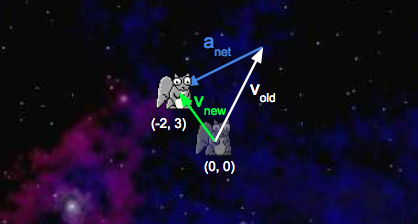For math/physics experts: You may be tempted to write a more accurate simulation where the force gradually increases over the specified time window. Don’t! Your simulation must follow exactly the rules above.

Write a method update(dt, fX, fY) that uses the steps above to update the body’s position and velocity instance variables (this method does not need to return anything).

Once you’re done, recompile and test your method with:

javac Body.java TestUpdate.java
java TestUpdate


Once you’ve done this, you’ve finished implementing the physics. Hoorah! You’re halfway there.

## (Optional) Testing Your Body Class

As the semester progresses, we’ll be giving you fewer and fewer tests, and it will be your responsibility to write your own tests. Writing tests is a good way to improve your workflow and be more efficient.

Go ahead and try writing your own test for the Body class. Make a TestBody.java file and write a test that creates two bodies and prints out the pairwise force between them. This is optional and we will not be grading this part of the assignment.

## Getting Started with the Simulator (NBody.java)

Create a file named NBody.java. NBody is a class that will actually run your simulation. This class will have NO constructor. The goal of this class is to simulate a universe specified in one of the data files. For example, if we look inside data/planets.txt (using the command line more command), we see the following:

#### When I run the simulation, my planets start rotating, but then quickly accelerate and disappear off of the bottom left of the screen.

• Look at the way you’re calculating the force exerted on a particular planet in one time step. Make sure that the force doesn’t include forces that were exerted in past time steps.
• Make sure you did not use Math.abs(...) when calculating calcForceExertedByX(...) and calcForceExertedByY(...). Also ensure that you are using a double to keep track of summed forces (not int)!

#### What is a constructor? How do I write one?

A constructor is a block of code that runs when a class is instantiated with the new keyword. Constructors serve the purpose of initializing a new object’s fields. Consider an example below:

public class Dog {
String _name;
String _breed;
int _age;

public Dog(String name, String breed, int age) {
_name = name;
_breed = breed;
_age = age;
}
}


The Dog class has three non-static fields. Each instance of the Dog class can have a name, a breed, and an age. Our simple constructor, which takes three arguments, initializes these fields for all new Dog objects.

#### I’m having trouble with the second Body constructor, the one that takes in another Body as its only argument.

Let’s walk through an example of how a constructor works. Suppose you use the Dog constructor above to create a new Dog:

Dog fido = new Dog("Fido", "Poodle", 1);


When this line of code gets executed, the JVM first creates a new Dog object that’s empty. In essence, the JVM is creating a “box” for the Dog, and that box is big enough to hold a box for each of the Dog’s declared instance variables. This all happens before the constructor is executed. At this point, here’s how you can think about what our new fluffy friend fido looks like (note that this is a simplification! We’ll learn about a more correct view of this when we learn about Objects and pointers later this semester):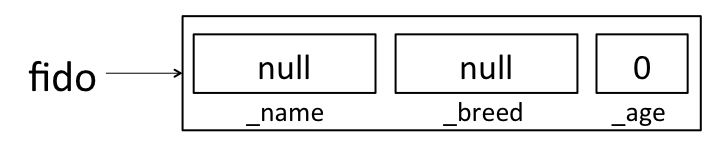Java will put some default values in each instance variable. We’ll learn more about where these defaults come from (and what null means) later this semester. For now, just remember that there’s space for all of the instance variables, but those instance variables haven’t been assigned meaningful values yet. If you ever want to see this in action, you can add some print statements to your constructor:

public Dog(String name, String breed, int age) {
System.out.println("_name: " + _name + ", _breed: " + _breed + ", _age: " + _age);
_name = name;
_breed = breed;
_age = age;
}


If this constructor had been used to create fido above, it would have printed:

_name: null, _breed: null, _age: 0


OK, back to making fido. Now that the JVM has made some “boxes” for fido, it calls the Dog constructor function that we wrote. At this point, the constructor executes just like any other function would. In the first line of the constructor, _name is assigned the value name, so that fido looks like:When the constructor completes, fido looks like:Now, suppose you want to create a new Dog constructor that handles cross-breeding. You want the new constructor to accept a name, an age, and two breeds, and create a new Dog that is a mixture of the two breeds. Your first guess for how to make this constructor might look something like this:

public Dog(String name, String breed1, String breed2, int age) {
Dog dog = new Dog(name, breed1 + breed2, age);
}


However, if you try to create a new Dog using this constructor:

Dog tommy = new Dog("Tommy", "Poodle", "Golden Retriever", 1);


This won’t do what you want! As above, the first thing that happens is that the JVM creates empty “boxes” for each of tommy’s instance variables:But then when the 4-argument constructor got called, it created a second Dog and assigned it to the variable dog. It didn’t change any of tommy’s instance variables. Here’s how the world looks after the line in our new constructor finishes:dog isn’t visible outside of the constructor method, so when the constructor completes, dog will be destroyed by the garbage collector (more on this later!) and all we’ll have is the still un-initialized tommy variable.

Here’s a cross-breed constructor that works in the way we’d like:

public Dog(String name, String breed1, String breed2, int age) {
this(name, breed1 + breed2, age);
}


Here, we’re calling the old 3-argument constructor on this; rather than creating a new Dog, we’re using the 3-argument constructor to fill in all of the instance variables on this dog. After calling this new constructor to create tommy, tommy will correctly be initialized to: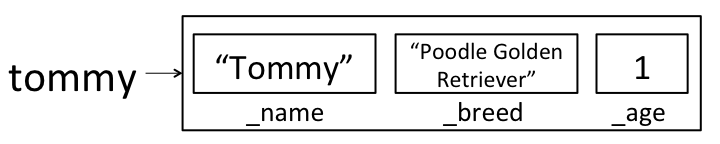We could have also written a new constructor that assigned each instance variable directly, rather than calling the existing constructor:

public Dog(String name, String breed1, String breed2, int age) {
_name = name;
_breed = breed1 + breed2;
_age = age;
}


## Acknowledgements

This assignment is a major revision by Josh Hug, Matthew Chow, and Daniel Nguyen of an assignment created by Robert Sedgewick and Kevin Wayne from Princeton University.